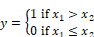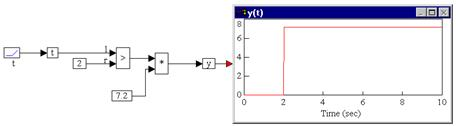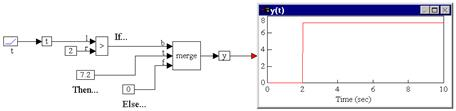## greater than (>)Block Category: Boolean

Inputs: Real, complex, or fixed-point scalars, or vectors or matrices.

On the input connector tabs, “l” represents the x1 and “r” represents the x2.

Description: The > block is useful in evaluating the Boolean > inequality. The output of the > block is 1 if and only if input signal x1 is less than input signal x2; otherwise the output is zero. On the connector tabs, “l” represents x1 and “r” represents x2.

Right-click the > block to assign a different function to the block.

#### Examples

1. Simple if-then-else construct

Consider a variable y such that:

If t > 2 then y = 7.2; else y = 0

Assume that t is simulation time realized as:By multiplying a constant value of 7.2 with the output of the > block, y is guaranteed to assume a value of 0 until the inequality is true. When the inequality is true, y assumes a value equal to the output of the * block.

2. Modified if-then-else construct

Using the above equation, it can also be realized as:The key difference in implementation is the use of a merge block rather than a * block. The merge block explicitly depicts the if-then-else structure, whereas the * block is a shortcut and can lead to confusion.